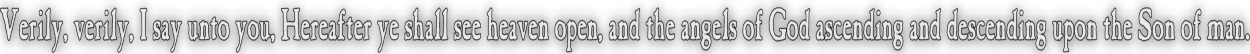None:
Polyps:
Strongs:MetaphysicsGödel's Modal Proof (Sometimes referred to as his ontological argument) This proof is the most complicated so far, including much notation. This is a somewhat shortened version of an online page for Gödel's proof, which can be visited HERE if you prefer to delve in. A nice neat .pdf file with the complete proof is found at this link. Postulates: N(a)=>a a=>P(a) N(a=>b) =>[ N(a)=>N(b)]  (modal modus ponens) N(a) is true whenever a is provable, (Necessitation postulate)  N(a)=>N(N(a))  Beckers Postulate S4 P(a)=>N(P(a))     Beckers postulate  S5 Definition F is an essence of x if for every property G of x,  N(G(x)) ó N(Fx=>Gx) I.e. N(Fx), F is an essential property of x Anselm's Axiom 1 P(a)  Anselm's Axiom 2 a=>N(a) Axiom G0 Pos(F)=>N(Pos(F)), I.e. positivity is free from contingency, And a form of 'pure attribution'. That is, what is not necessary could not be positive. Axiom G1 Pos(F)=>¬pos(¬F)  Negation of a positive property is privation, i.e. its lack could not be positive. Definition F => H   if   N(given x)[Fx=>Hx] and we say F entails the property of  H necessarily in x, that is, x necessarily has H if it has F. Axiom G2 Pos(F)=>[(F=>H)=>Pos(H)] A property entailed from a positive is itself positive. Privation of H means lack of F. Definition F is consistent if it is possibly exemplified, ie,  P(\$x)F(x) is true. Theorem G1 : Pos(F)=>F is consistent Let Pos(F) be true, if F is inconsistent, then F=>¬F. So G2 implies Pos(¬F) is true, but from G1 and Pos(F), ¬pos(¬F), a contradiction, QED. Definition G1 x is 'God-like', ie G(x) is true, if all essential properties of x are positive, and all positive properties of x are as essential properties, ie G(x) ó ("F):[N(Fx) ó Pos(F)] Definition G2 We formulate an essence of an individual as follows, F Ess x = (\$F)("H) [ N(Hx) ó (F=>H) ] Thus F and H will be realized in common or fail to be realized in common as essences. Definition G3 NE(x) ó ("F):[F Ess x => N(\$y)Fy] We say that an individual x exists necessarily if every property which is an essence of x is necessarily realized in some individual Axiom G3 Pos(G) Being God-like is positive. Corollary G1 P(\$x)(Gx) Proof : theorem G1, Axiom G3 Axiom G4 Pos(NE) necessary existence is positive Theorem G2 If a being is God-like, then being God-like is an essence of the individual. Gx  =>  G Ess x Proof Suppose Gx is true and x necessarily has property H., Ie N(Hx) is true. Then by definition G1, we have Pos(H), but N[Pos(H) => ( \$y)(Gy => Hy)] from definition G1 (Recall that Gx is true) and that if something necessarily has a property then it has the property. However, by Axiom G0, N(Pos(H)) is true, by modal modus ponens, we have N(\$y)(Gy=>Hy). So if x has any property H necessarily, then that is entailed by the property G, so G=>H. Conversely, suppose Gx is true and G=>H then by axioms G2 and G3, we have Pos(H) ). It follows that a God-like individual x has property H necessarily, by Definition G1. That is, N(Hx) is true Putting both sides together, G Ess x Theorem G3 Necessarily the property of being Godlike is exemplified N(\$x)Gx Proof IF Gx were true, then by Definition G1 x has every positive property necessarily. Axiom G4 states necessary existence is positive, so NE(x) is true. But by theorem G2 if Gx were true, G Ess x is true by Definition G3, if any x is godlike then 'godlike' as a property is necessarily exemplified, ie (\$x)Gx => N(\$x)Gx this follows from Definition G1, Axiom G4 and Theorem G2. This is axiom 2 of Anselms argument. The necessitation axiom of modal logic gives N[(\$x)Gx =>N(\$x)Gx] A theorem of modal logic (provable : consider (a=>b) = N¬(a&¬b)) is that; N(p=>q) => (N(p)=>N(q)) Combining this, P(\$x)Gx => P(N(\$x)Gx) By corollary G1, ie P(\$x)Gx is true, it follows that P(N((\$x)Gx)) is also true. But by a theorem of S5, (simple contrapositive shows true) P(N(x))=>N(x) Thus N(\$x)Gx So it is necessary that such an individual exists who has the property of being 'God-like'. Which was what was wanted. //Continue To Next Page Return To Section Start Return To Previous Page'